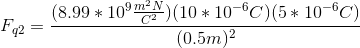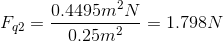# AP Physics B : Using Coulomb's Law

## Example Questions

### Example Question #1 : Coulomb's Law

Charges A and B are placed a distance of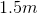from one another. The charge of particle A is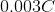whereas the charge of particle B is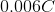. Charge B experiences an electrostatic force of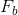from charge A. Similarly, charge A experiences an electrostatic force of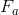from charge B.

What is the ratio ofto?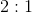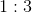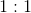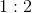Explanation:

This question is very simple if you realize that the force experienced by both charges is equal.

The definition of the two electrostatic forces are given by Coulomb's law: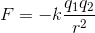In this question, we can rewrite this equation in terms of our given system.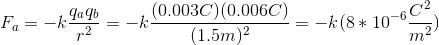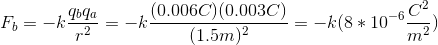It doesn’t matter if the charges of the two particles are different; both particles experience the same force because the charges of both particles are accounted for in the electrostatic force equation (Coulomb's law). This conclusion can also be made by considering Newton's third law: the force of the first particle on the second will be equal and opposite the force of the second particle on the first.

Since the forces are equal, their ratio will be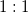.

### Example Question #2 : Coulomb's Law

An excess charge of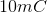is put on an ideal neutral conducting sphere with radius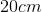. What is the Coulomb force this excess charge exerts on a point charge of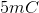that is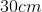from the surface of the sphere?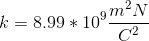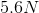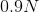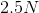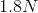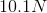Explanation:

Two principal realizations help with solving this problem, both derived from Gauss’ law for electricity:

1) The excess charge on an ideal conducting sphere is uniformly distributed over its surface

2) A uniform shell of charge acts, in terms of electric force, as if all the charge were contained in a point charge at the sphere’s center

With these realizations, an application of Coulomb’s law answers the question. If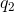is the point charge outside the sphere, then the force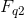onis: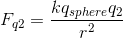In this equation,is Coulomb’s constant,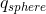is the excess charge on the spherical conductor, andis total distance in meters offrom the center of the conducting sphere.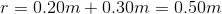Using the given values in this equation, we can calculate the generated force: